## Design and Theory

Y-Dimension is the distance from the end of the part to the first hole.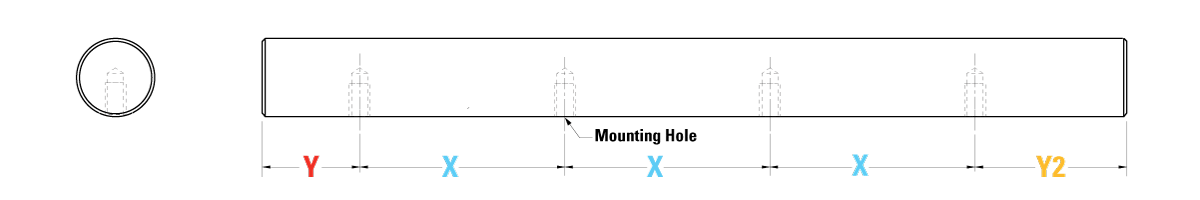#### Predrilled 60 case shafting

Y - The end of the shaft to the first hole

Y2 - The last hole to the end of the shaft.

X – Spacing between the holes (Sometimes called distance on centers)

The Y-dimension applies to just about any linear rail, shaft or assembly with mounting holes.  Below are shown several examples which are only a few of the products where the Y-dimension applies.

Knowing what the Y-dimension is necessary to ensure the holes in the mating mounting surface are drilled at the correct locations.  When ordering a replacement part it is necessary to ensure the parts will be the same.  The Thomson standard is to have the Y-dimension equal on both ends but special Y-dimensions are common.

The following will describe the Y-dimension in more detail.
For illustration purposes of explaining the Y-dimension, we will show an example piece of material longer than our needs with the part we will cut out will be highlighted with an orange box.  The left edge of the box is where the first cut will be and the right edge of the box is where the second cut would be.  See illustration below:

The easiest way to understand the Y-dimension may be to look at an example.  In this example we will look at size 25 profile rail.  Below is an image from the catalog.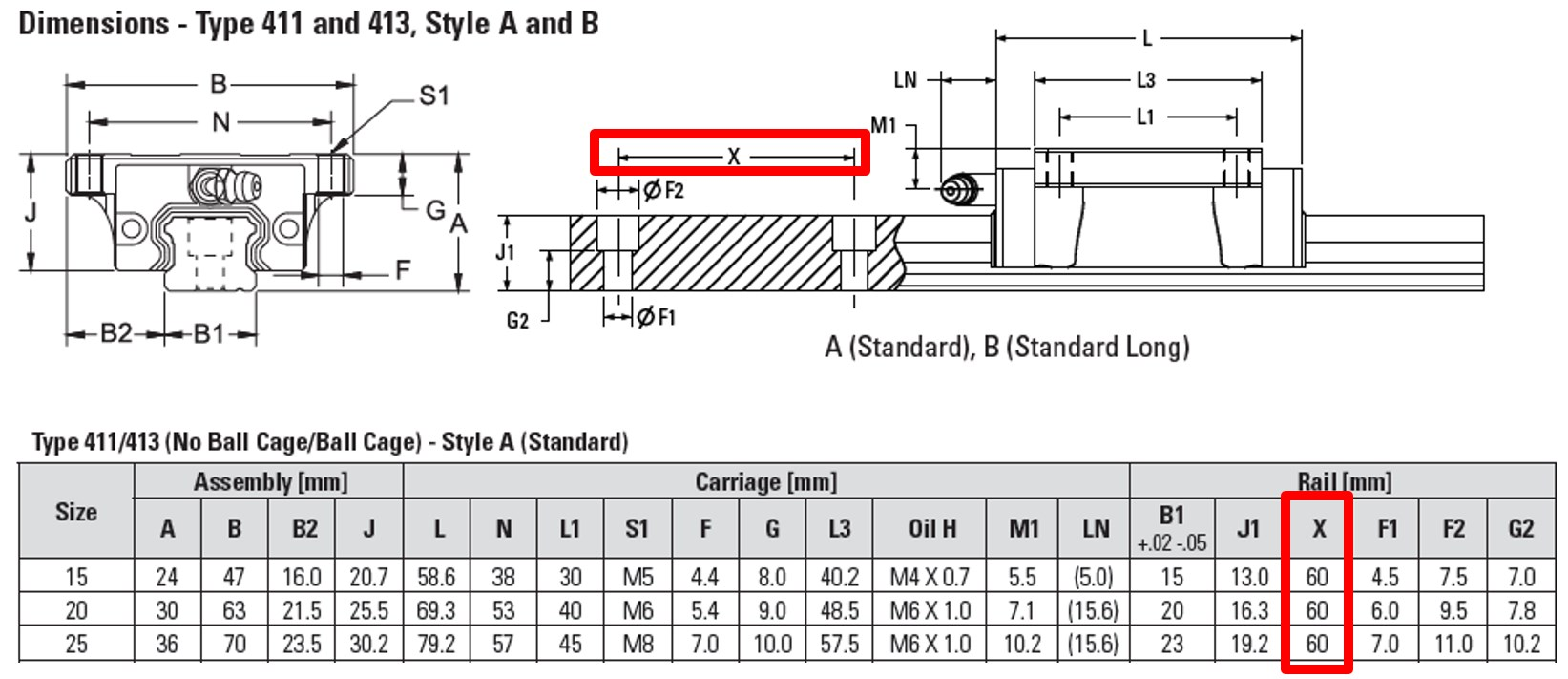#### Catalog page

In the catalog we can find the X-dimension of the size 25 profile rail is 60mm, highlighted above in red.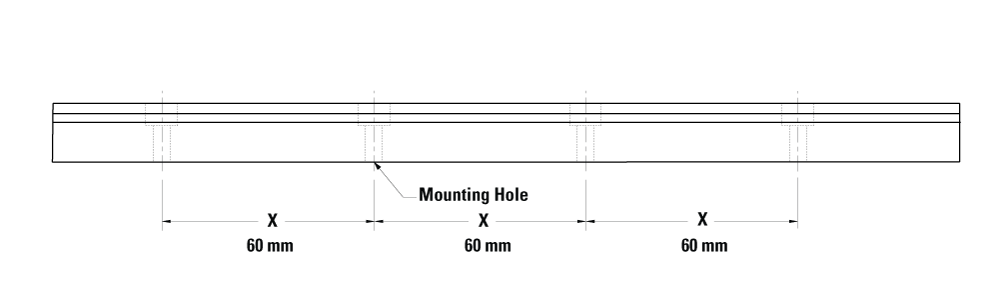#### Rail with X-dimension

Above is our sample rail with the X-dimensions added.  Now if we want a rail that is 200 mm long where do we cut?  If we choose to make the first cut 5mm from the first hole (Y=5 mm) then measure down the rail 200 mm and make the second cut, we then have a 200 mm long piece of rail.  See below for an illustration.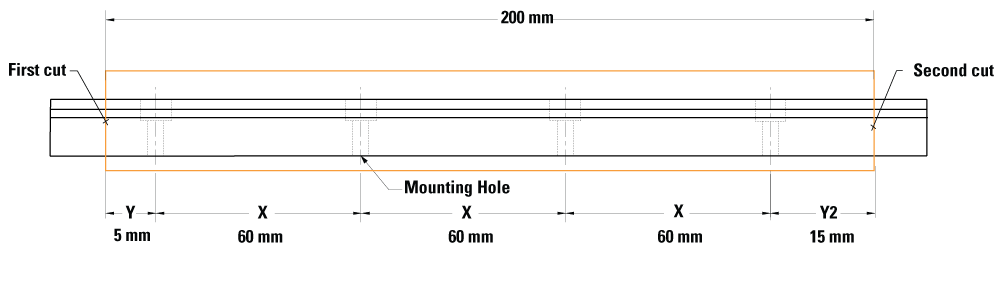#### Example 200 mm long with Y = 5 mm

In this example Y2 turns out to be 15 mm.  Here is how the math works:
• There are three “X dimension” sections at 60 mm each or 180 mm
• Total length wanted is 200 mm, subtract 180 mm (between the X’s) 200 – 180 = 20 mm
• This means there is 20 mm to be split between Y and Y2. Y=5 mm so 20 - 5 = 15 mm = Y2
The Thomson standard is for Y to equal Y2 and in this case they aren't equal.  If we took the 20 mm that needs to be split between Y and Y2 and divide it equally Y and Y2 would both equal 10 mm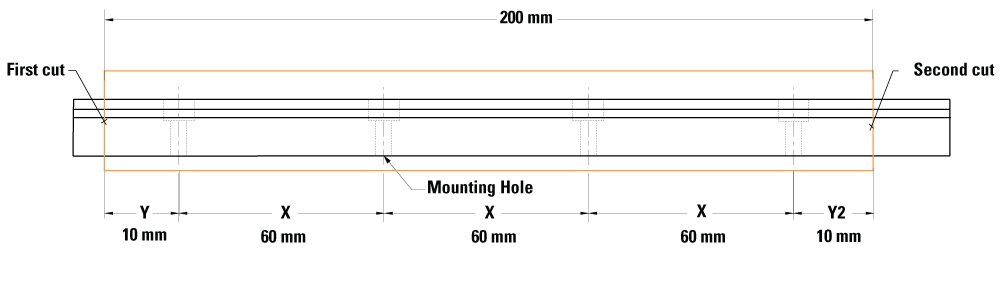#### Example 200 mm long with Y = Y2 = 10 mm

Here is how the math works:
• There are three “X dimension” sections at 60mm each or 180 mm
• Total length wanted is 200 mm, subtract 180mm (between the X’s) 200 – 180 = 20 mm
• This means there is 20mm to be split between Y and Y2 or 20 mm / 2 = 10 mm = Y = Y2
Seems easy enough?  Suppose we want 190 mm and Y = 10 mm, what would happen.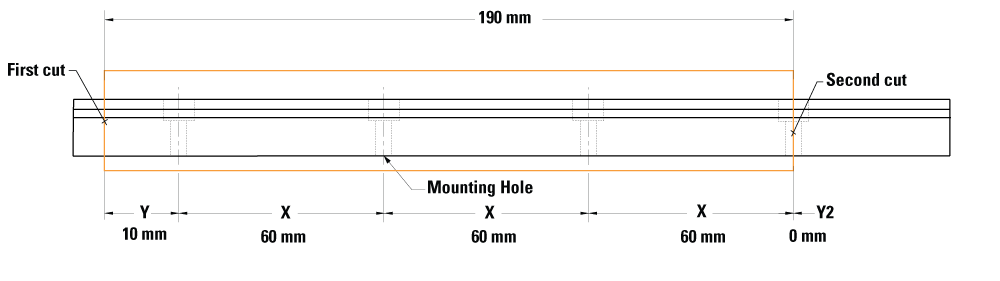#### Example 190 mm long with Y = 10 mm

If we make the first cut at 10 mm from the hole, then measure down 190 mm and make the second cut we cut right through a hole (Y2 = 0).  Below is the math.
• There are three “X dimension” sections at 60mm each or 180 mm
• Total length wanted is 190 mm, subtract 180mm (between the X’s) 190 – 180 = 10 mm
• This means there is 10mm to be split between Y and Y2. Y = 10 mm so Y2 = 10 - 10 = 0 mm
Cutting through a hole is not usually wanted and generally is avoided.  If we were to use the standard Y (Y = Y2) what would it be?

It's easy right?  Take the 10 mm left and split it evenly between Y and Y2 making them 5 mm each.  Unfortunately the hole radius of 5.5 mm and that means a Y of 5 mm would cut into the hole on both ends. So we can't use a Y = 5 mm without cutting a hole.  There just isn't a way to fit three X-dimensions into our 190 mm rail, We have to use only two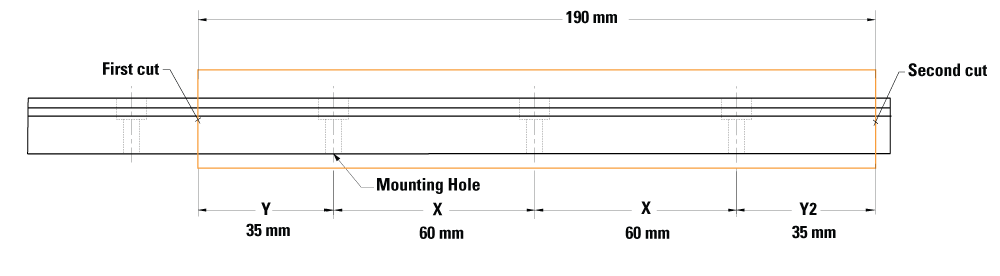#### Example 190 mm long with Y = Y2 mm

Using two X-dimensions we can do the calculation again. Here is how the math works:
• There are two “X dimension” sections at 60mm each or 120 mm
• Total length wanted is 190 mm, subtract 120mm (between the X’s) 190 – 120 = 70 mm
• This means there is 70mm to be split between Y and Y2 or 70 / 2 = 35 mm.
Perfect.